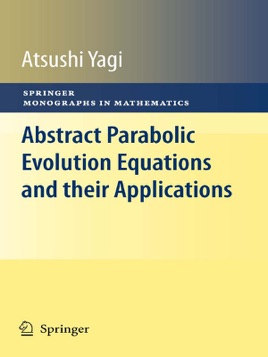• USD 99.99

## Descripción de editorial

The semigroup methods are known as a powerful tool for analyzing nonlinear diffusion equations and systems. The author has studied abstract parabolic evolution equations and their applications to nonlinear diffusion equations and systems for more than 30 years. He gives first, after reviewing the theory of analytic semigroups, an overview of the theories of linear, semilinear and quasilinear abstract parabolic evolution equations as well as general strategies for constructing dynamical systems, attractors and stable-unstable manifolds associated with those nonlinear evolution equations.

In the second half of the book, he shows how to apply the abstract results to various models in the real world focusing on various self-organization models: semiconductor model, activator-inhibitor model, B-Z reaction model, forest kinematic model, chemotaxis model, termite mound building model, phase transition model, and Lotka-Volterra competition model. The process and techniques are explained concretely in order to analyze nonlinear diffusion models by using the methods of abstract evolution equations.

Thus the present book fills the gaps of related titles that either treat only very theoretical examples of equations or introduce many interesting models from Biology and Ecology, but do not base analytical arguments upon rigorous mathematical theories.